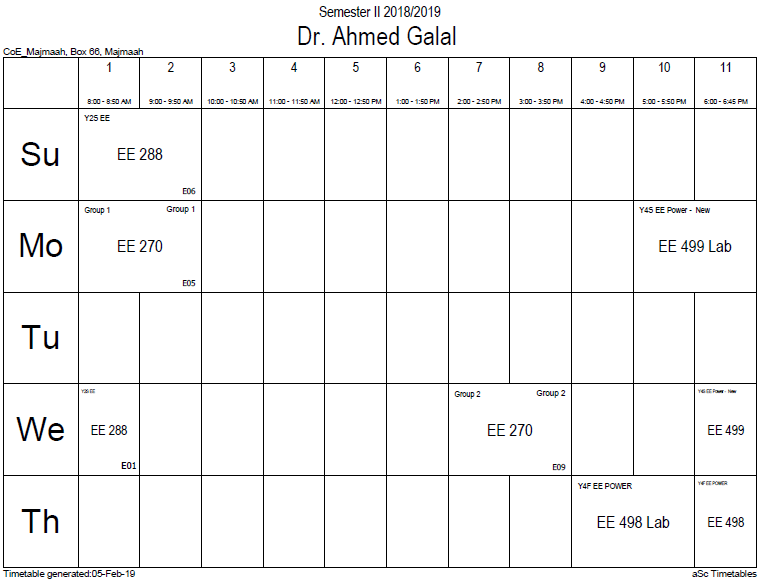## H-field and magnet

The H-field is defined as:$mathbf{H} equiv frac{mathbf{B}}{mu_0}-mathbf{M},$(definition of H in SI units)

With this definition, Ampere's law becomes:$oint mathbf{H} cdot doldsymbol{ell} = oint (frac{mathbf{B}}{mu_0} - mathbf{M}) cdot doldsymbol{ell} = I_{mathrm{tot}}- I_{mathrm{b}} = I_{mathrm{f}},$

where If represents the 'free current' enclosed by the loop so that the line integral of H does not depend at all on the bound currents. For the differential equivalent of this equation see Maxwell's equations. Ampere's law leads to the boundary condition$H_{1,parallel} - H_{2,parallel} = mathbf{K}_ ext{f},$

where Kf is the surface free current density.

Similarly, a surface integral of H over any closed surface is independent of the free currents and picks out the 'magnetic charges' within that closed surface:$oint_S mu_0 mathbf{H} cdot mathrm{d}mathbf{A} = oint_S (mathbf{B}- mu_0 mathbf{M})cdot mathrm{d}mathbf{A}= (0 - (-q_M)) = q_M,$

which does not depend on the free currents.

The H-field, therefore, can be separated into two independent parts:$mathbf{H} = mathbf{H}_0 + mathbf{H}_d, ,$

where H0 is the applied magnetic field due only to the free currents and Hd is the demagnetizing field due only to the bound currents.

The magnetic H-field, therefore, re-factors the bound current in terms of 'magnetic charges'. The H field lines loop only around 'free current' and, unlike the magnetic B field, begins and ends near magnetic poles as well.

### Office HoursNo office hours### My Timetable### Contactsemail: [email protected]

Phone: 2570

### Welcome

Welcome To Faculty of Engineering### IEEEhttp://www.ieee.org/

/

### Bookmarkshttp://www.utk.edu/research/

http://science.doe.gov/grants/index.asp

http://www1.eere.energy.gov/vehiclesandfuels/

http://www.eere.energy.gov/

### Upcoming Conferences### Engineering quotes### Travel Web Siteshttp://www.hotels.com/

http://www.orbitz.com/

http://www.hotwire.com/us/index.jsp

http://www.kayak.com/

### Blackboardستقام اختبارات الميدتيرم يوم الثلاثاء 26-6-1440

حسب الجدول المعلن بلوحات الاعلان

### Summer trainingThe registration for summer training will start from 5th week of second semesterClass registration week 1

### برنامج التجسير### إحصائية الموقع

عدد الصفحات: 2879

البحوث والمحاضرات: 1280

الزيارات: 73005